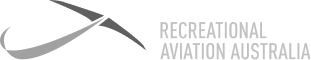# Definition: Lift Formula

Lift = CL x ½ p V2 (squared) S CL is the co-efficient of lift (AoA) p (rho) is the air density V (velocity) The airspeed S is the surface area of the wing In simple terms, L = AoA x Airspeed### Lift Formula

Lift = CL x ½ p V2 (squared) S CL is the co-efficient of lift (AoA) p (rho) is the air density V (velocity) The airspeed S is the surface area of the wing In simple terms, L = AoA x Airspeed

7 Day

FREE

Trial